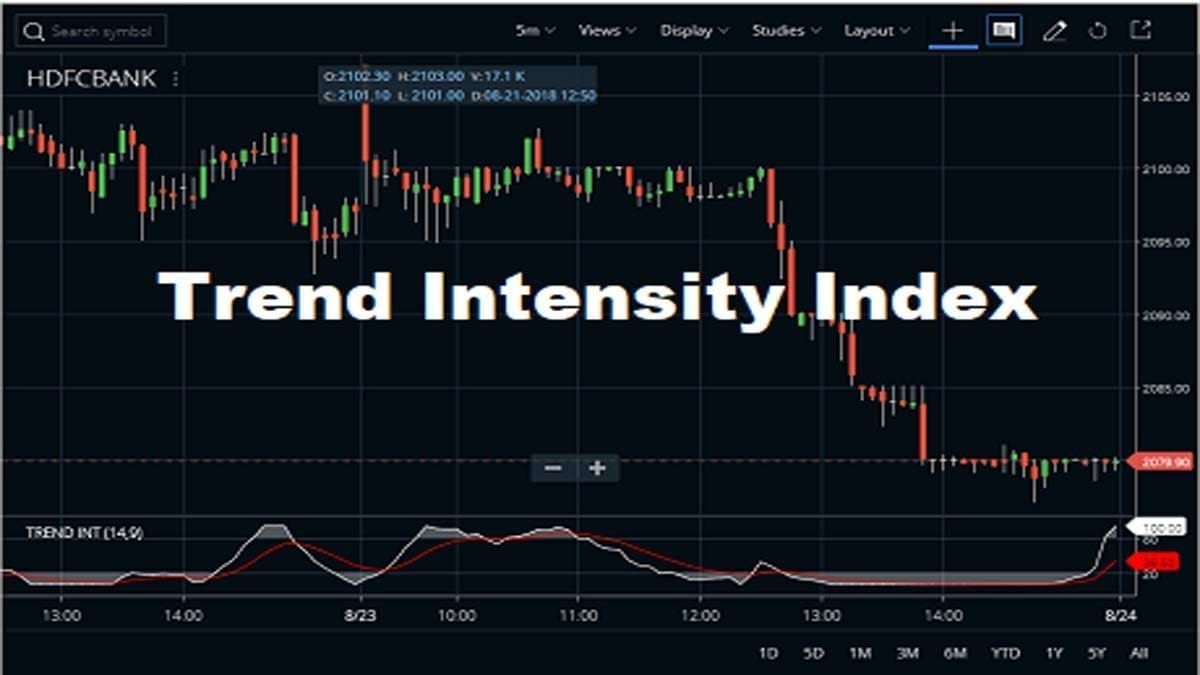# Trend Intensity Index Indicator Strategy, FormulaTrend Intensity Index indicator was developed by M. H. Pee. This indicator is used to measure the strength of a trend of a sock, ETF index or any other tradable security. The RSI principles of calculation applied to the closing price deviations instead of the closing prices are uses Trend Intensity Index (TII) indicator. In this TII indicator is used to generate trading signals on assumption that the stronger current trend is the more analyzed stock like index, ETF, etc. which will continue moving in the direction of the current trend.

## How To Apply Trend Intensity Index Indicator On Chart?

If traders can know more about the TII indicator, then they can find it in the STUDIES section of Zerodha Kite. It is also available in Kite mobile App. The default Field is Close and you can set this to open, High, Low, etc. The default Period is 14 and the Signal Period is 9. You can set and change these two values also. You can also check the image below to understand how to attach the TII indicator in HDFC Bank share price chart. Use this indicator on to any charts likes daily time frame, weekly time frame, monthly time frame or intraday time frame.

## Formula and Calculations of Trend Intensity Index Indicator

The calculation of Trend Intensity Index (TII) indicator which is applying by Relative Strength Index (RSI) principles to the closing price deviations.

• Simple Moving Average (SMA) of the closing prices:
SMA = SMA (Close, n). where n is SMA bar period.
• Positive and Negative deviation of the closing prices from SMA calculation :
Dev = Close – SMAIF Dev is greater than 0 then posDev equals to Dev.IF Dev is less than 0 then negDev equals to abs(Dev).
• The total of Positive and Negative deviations for the period is twice smaller than the period used for the SMA calculation :
1. SDpos = sum(posDev, m).
2. SDneg = sum(negDev, m).
3. here, m is period twice smaller than n : m = n/2. If the bar period number (n) is not even then m equals to (n+1)/2.
• Trend Intensity index :
TII = 100 * (SDpos) / (SDpos + SDneg).
• 2nd line or signal line as EMA applied to Trend Intensity Index: Signal Line = EMA(TII, k), where k is bar period of EMA applied to TII.

## Conclusion:

Like other oscillators, the TII is also bounded between 0 and 100. When the indicator shows a reading above 50, this indicates a bull trend. When the TII shows a reading below 50, a bear trend is probably present. The TII distances from the 50 level which is the greater the intensity of the current trend. The closer the Trend Intensity Index indicator is to one hundred, the stronger the bull trend. The closer this indicator is to zero, the stronger the bear trend.

NEW TO STOCK MARKET?## READ THIS FREE EBOOK TO LEARN...

• 4 rules of making millions in the stock market.
• How to retire as a multi millionaire.## Author: Ankita Chakraborty

Ankita has done her Diploma Engineering in Computer Science & Technology. She is pursuing her degree in Engineering and also well experienced in the equity market and real estate related content writing. She is the one who has developed the technical indicators section of our site.

## 2 thoughts on “Trend Intensity Index Indicator Strategy, Formula”

1.Tithal Shah says:

Thanks for developing such a nice Indicator

2.Tithal Shah says:

Very perfect indicator for getting signal of trading

This site uses Akismet to reduce spam. Learn how your comment data is processed.